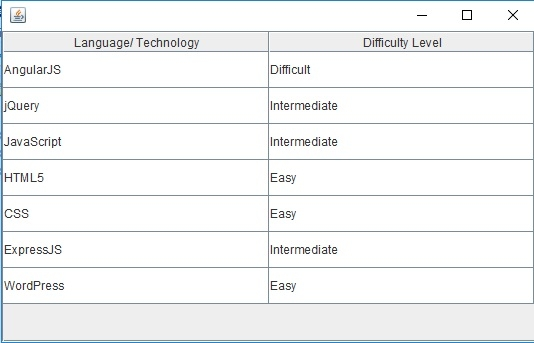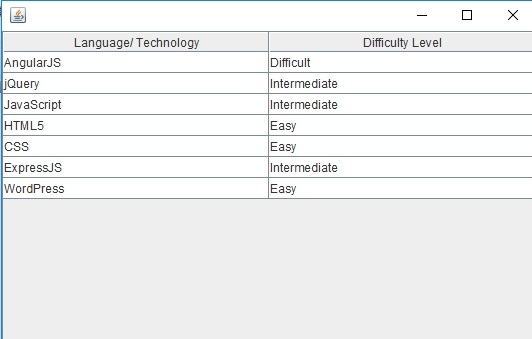# Java Program to increase the row height in a JTable

To increase the row height, use the setRowHeight() method for a table in Java. Row height is the height of the row in pixels.

Let’s say the following is our table −

DefaultTableModel tableModel = new DefaultTableModel();
JTable table = new JTable(tableModel);

Set the rows and columns and increase the row height by first getting the current height and incrementing it. We are incrementing by 20 pixels here −

table.setRowHeight(table.getRowHeight() + 20);

The following is an example to increase the row height −

## Example

package my;
import javax.swing.JFrame;
import javax.swing.JScrollPane;
import javax.swing.JTable;
import javax.swing.table.DefaultTableModel;
public class SwingDemo {
public static void main(String[] argv) throws Exception {
DefaultTableModel tableModel = new DefaultTableModel();
JTable table = new JTable(tableModel);
tableModel.insertRow(0, new Object[] { "CSS", "Easy" });
tableModel.insertRow(0, new Object[] { "HTML5", "Easy"});
tableModel.insertRow(0, new Object[] { "JavaScript", "Intermediate" });
tableModel.insertRow(0, new Object[] { "jQuery", "Intermediate" });
tableModel.insertRow(0, new Object[] { "AngularJS", "Difficult"});
tableModel.insertRow(tableModel.getRowCount(), new Object[] {"ExpressJS", "Intermediate" });
// appending a new row
tableModel.addRow(new Object[] { "WordPress", "Easy" });
// set row height
table.setRowHeight(table.getRowHeight() + 20);
JFrame f = new JFrame();
f.setSize(550, 350);
f.setVisible(true);
}
}

The output is as follows. Here we have set the row height to 20 −Now, let us change the row height to 5 and spot the difference −Updated on: 30-Jul-2019

734 Views